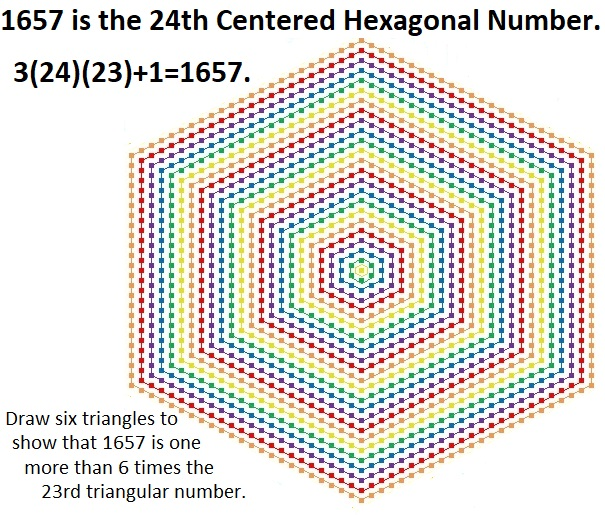# Prime Number 1657 is the 24th Centered Hexagonal Number

Contents

### Today’s Puzzle:

Draw six triangles on the graphic below to show that 1657 is one more than 6 times the 23rd triangular number.### Factors of 1657:

• 1657 is a prime number.
• Prime factorization: 1657 is prime.
• 1657 has no exponents greater than 1 in its prime factorization, so √1657 cannot be simplified.
• The exponent in the prime factorization is 1. Adding one to that exponent we get (1 + 1) = 2. Therefore 1657 has exactly 2 factors.
• The factors of 1657 are outlined with their factor pair partners in the graphic below.How do we know that 1657 is a prime number? If 1657 were not a prime number, then it would be divisible by at least one prime number less than or equal to √1657. Since 1657 cannot be divided evenly by 2, 3, 5, 7, 11, 13, 17, 19, 23, 29, 31, or 37, we know that 1657 is a prime number.

### More About the Number 1657:

1657 is the sum of two squares:
36² + 19² = 1657.

1657 is the hypotenuse of a primitive Pythagorean triple:
935-1368-1657, calculated from 36² – 19², 2(36)(19), 36² + 19².

Here’s another way we know that 1657 is a prime number: Since its last two digits divided by 4 leave a remainder of 1, and 36² + 19² = 1657 with 36 and 19 having no common prime factors, 1657 will be prime unless it is divisible by a prime number Pythagorean triple hypotenuse less than or equal to √1657. Since 1657 is not divisible by 5, 13, 17, 29, or 37, we know that 1657 is a prime number.

Do you notice anything else special about the number 1657 in this color-coded chart?This site uses Akismet to reduce spam. Learn how your comment data is processed.